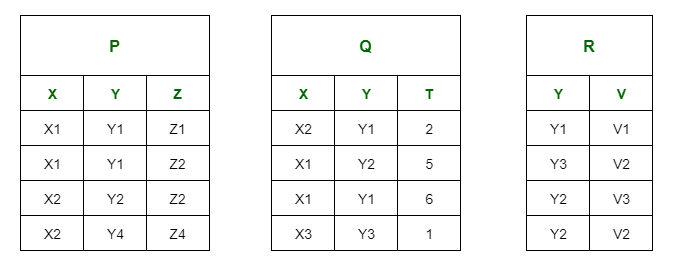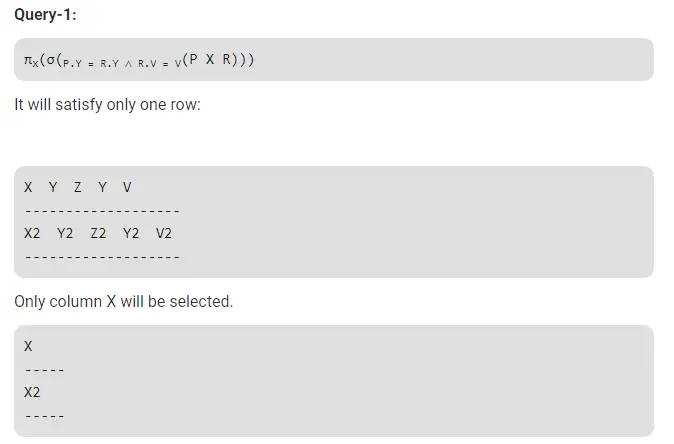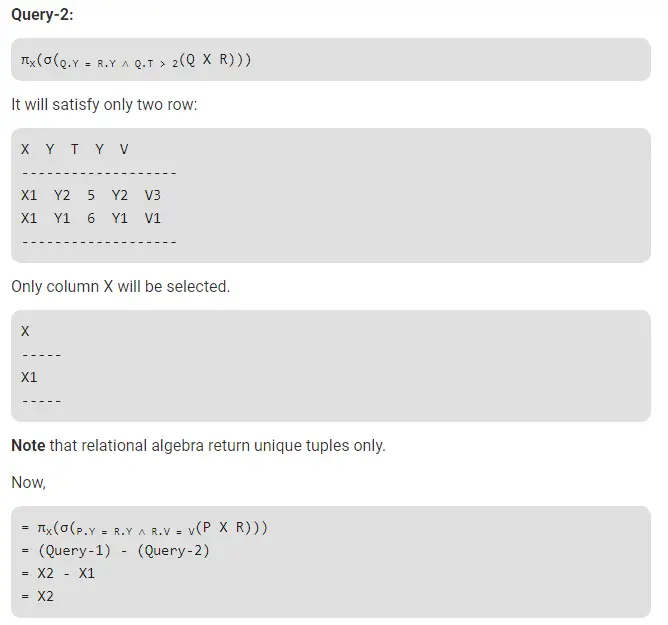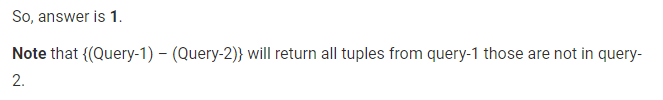# Gate CS-2019 Question Paper With Solutions

Q. 63 Consider the following relation P(X, Y, Z), Q(X, Y, T) and R(Y, V):How many tuples will be returned by the following relational algebra query?

`πx(σ(P.Y = R.Y ∧ R.V = V(P X R))) - πx(σ(Q.Y = R.Y ∧ Q.T > 2(Q X R)))`

Note: This was Numerical Type question.

(A) 3

(B) 1

(C) 2

(D) 4